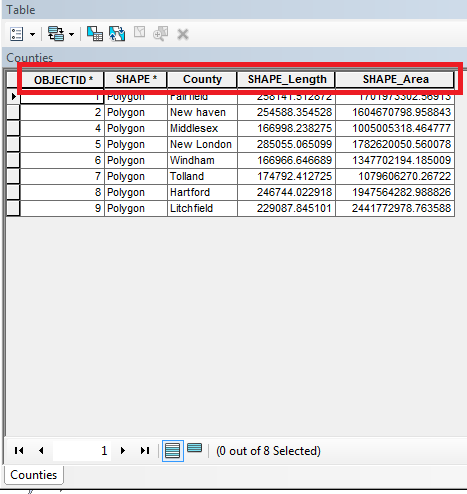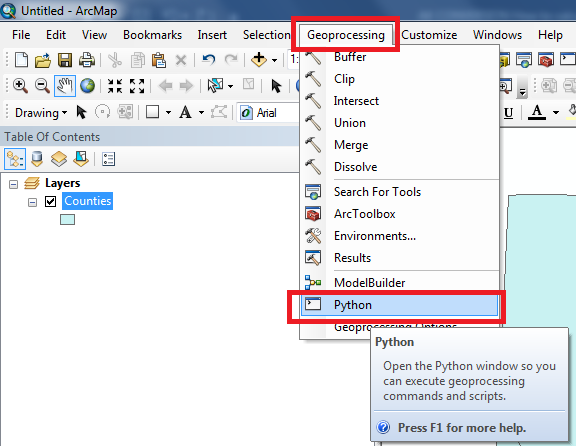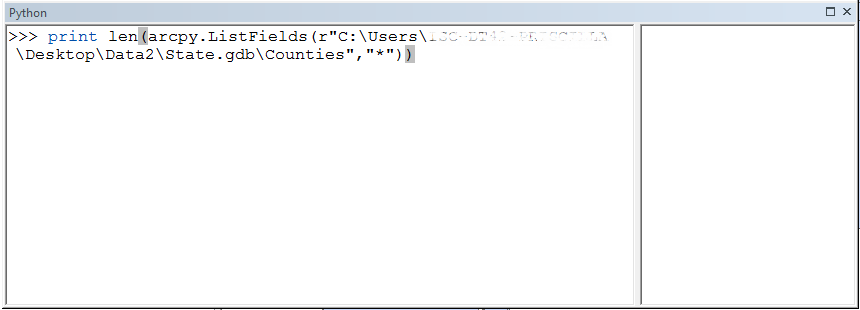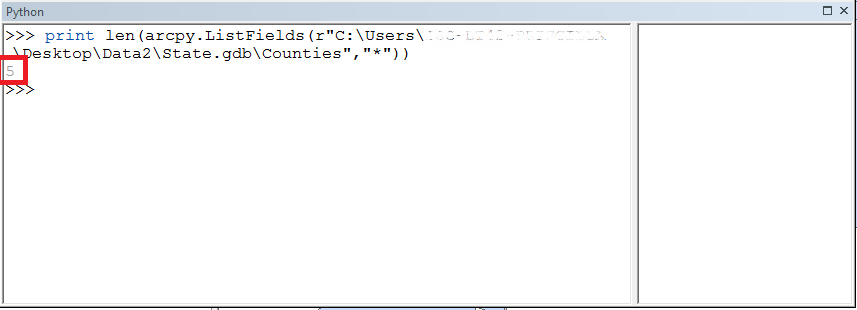HOW TO

# Calculate the total number of fields in a feature class using Python ListFields() in ArcMap

Last Published: April 25, 2020

## Summary

In ArcGIS Desktop, the Get Count geoprocessing tool is used to calculate the total number of rows in an attribute table. However, this tool does not calculate the total number of columns, which represent the total number of fields in a feature class.

Calculating the total number of fields is useful when there are a number of attributes in a feature class. The total can be derived using the Python ListFields() function.## Procedure

The workflow below describes how to calculate the total number of fields in a feature class using the Python ListFields() function.

1. In ArcMap, click the Geoprocessing tab, and select Python to open the Python command window.1. In the Python window, enter the Python ListFields() function.
```print len(arcpy.ListFields(r"location of your feature class or shapefile","*"))
```
```Note:

In this example, the following expression is used to count the fields in the Counties feature class:

```print len(arcpy.ListFields(r"C:\Users\*****\Desktop\Data2\State.gdb\Counties","*"))
```1. Place the cursor at the end of the expression and press Enter. The image below shows the total number of fields for the Counties feature class is 5.Article ID:000022586

Software:
• ArcMap

Get help from ArcGIS experts

Contact technical support